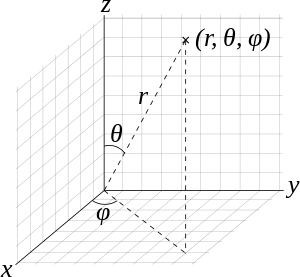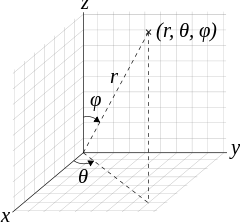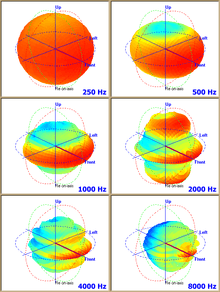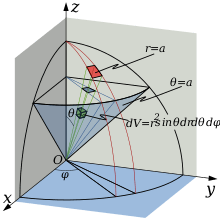# Spherical coordinate systemSpherical coordinates (r, θ, φ) as commonly used in physics (ISO convention): radial distance r, polar angle θ (theta), and azimuthal angle φ (phi). The symbol ρ (rho) is often used instead of r.Spherical coordinates (r, θ, φ) as often used in mathematics: radial distance r, azimuthal angle θ, and polar angle φ. The meanings of θ and φ have been swapped compared to the physics convention.

In mathematics, a spherical coordinate system is a coordinate system for three-dimensional space where the position of a point is specified by three numbers: the radial distance of that point from a fixed origin, its polar angle measured from a fixed zenith direction, and the azimuth angle of its orthogonal projection on a reference plane that passes through the origin and is orthogonal to the zenith, measured from a fixed reference direction on that plane. It can be seen as the three-dimensional version of the polar coordinate system.

The radial distance is also called the radius or radial coordinate. The polar angle may be called colatitude, zenith angle, normal angle, or inclination angle.

The use of symbols and the order of the coordinates differs between sources. In one system frequently encountered in physics (r, θ, φ) gives the radial distance, polar angle, and azimuthal angle, whereas in another system used in many mathematics books (r, θ, φ) gives the radial distance, azimuthal angle, and polar angle. In both systems ρ is often used instead of r. Other conventions are also used, so great care needs to be taken to check which one is being used.

A number of different spherical coordinate systems following other conventions are used outside mathematics. In a geographical coordinate system positions are measured in latitude, longitude and height or altitude. There are a number of different celestial coordinate systems based on different fundamental planes and with different terms for the various coordinates. The spherical coordinate systems used in mathematics normally use radians rather than degrees and measure the azimuthal angle counterclockwise from the x-axis to the y-axis rather than clockwise from north (0°) to east (+90°) like the horizontal coordinate system. The polar angle is often replaced by the elevation angle measured from the reference plane. Elevation angle of zero is at the horizon.

The spherical coordinate system generalises the two-dimensional polar coordinate system. It can also be extended to higher-dimensional spaces and is then referred to as a hyperspherical coordinate system.

## Definition

To define a spherical coordinate system, one must choose two orthogonal directions, the zenith and the azimuth reference, and an origin point in space. These choices determine a reference plane that contains the origin and is perpendicular to the zenith. The spherical coordinates of a point P are then defined as follows:

• The radius or radial distance is the Euclidean distance from the origin O to P.
• The inclination (or polar angle) is the angle between the zenith direction and the line segment OP.
• The azimuth (or azimuthal angle) is the signed angle measured from the azimuth reference direction to the orthogonal projection of the line segment OP on the reference plane.

The sign of the azimuth is determined by choosing what is a positive sense of turning about the zenith. This choice is arbitrary, and is part of the coordinate system's definition.

The elevation angle is 90 degrees (π/2 radians) minus the inclination angle.

If the inclination is zero or 180 degrees (π radians), the azimuth is arbitrary. If the radius is zero, both azimuth and inclination are arbitrary.

In linear algebra, the vector from the origin O to the point P is often called the position vector of P.

### Conventions

Several different conventions exist for representing the three coordinates, and for the order in which they should be written. The use of (r, θ, φ) to denote radial distance, inclination (or elevation), and azimuth, respectively, is common practice in physics, and is specified by ISO standard 80000-2:2009, and earlier in ISO 31-11 (1992).

However, some authors (including mathematicians) use φ for inclination (or elevation) and θ for azimuth, which "provides a logical extension of the usual polar coordinates notation". Some authors may also list the azimuth before the inclination (or elevation), and/or use ρ (rho) instead of r for radial distance. Some combinations of these choices result in a left-handed coordinate system. The standard convention (r, θ, φ) conflicts with the usual notation for the two-dimensional polar coordinates, where θ is often used for the azimuth. It may also conflict with the notation used for three-dimensional cylindrical coordinates. 

The angles are typically measured in degrees (°) or radians (rad), where 360° = 2π rad. Degrees are most common in geography, astronomy, and engineering, whereas radians are commonly used in mathematics and theoretical physics. The unit for radial distance is usually determined by the context.

When the system is used for physical three-space, it is customary to use positive sign for azimuth angles that are measured in the counter-clockwise sense from the reference direction on the reference plane, as seen from the zenith side of the plane. This convention is used, in particular, for geographical coordinates, where the "zenith" direction is north and positive azimuth (longitude) angles are measured eastwards from some prime meridian.

Major conventions
coordinates corresponding local geographical directions
(Z, X, Y)
right/left-handed
(r, θinc, φaz,right) (U, S, E) right
(r, φaz,right, θel) (U, E, N) right
(r, θel, φaz,right) (U, N, E) left
Note: easting (E), northing (N), upwardness (U). Local azimuth angle would be measured, e.g., counterclockwise from S to E in the case of (U, S, E).

### Unique coordinates

Any spherical coordinate triplet (r, θ, φ) specifies a single point of three-dimensional space. On the other hand, every point has infinitely many equivalent spherical coordinates. One can add or subtract any number of full turns to either angular measure without changing the angles themselves, and therefore without changing the point. It is also convenient, in many contexts, to allow negative radial distances, with the convention that (−r, θ, φ) is equivalent to (r, θ + 180°, φ) for any r, θ, and φ. Moreover, (r, −θ, φ) is equivalent to (r, θ, φ + 180°).

If it is necessary to define a unique set of spherical coordinates for each point, one must restrict their ranges. A common choice is:

r ≥ 0
0° ≤ θ ≤ 180° (π rad)
0° ≤ φ < 360° (2π rad)

However, the azimuth φ is often restricted to the interval (−180°, +180°], or (−π, +π] in radians, instead of [0, 360°). This is the standard convention for geographic longitude.

The range [0°, 180°] for inclination is equivalent to [−90°, +90°] for elevation (latitude).

Even with these restrictions, if θ is 0° or 180° (elevation is 90° or −90°) then the azimuth angle is arbitrary; and if r is zero, both azimuth and inclination/elevation are arbitrary. To make the coordinates unique, one can use the convention that in these cases the arbitrary coordinates are zero.

### Plotting

To plot a dot from its spherical coordinates (r, θ, φ), where θ is inclination, move r units from the origin in the zenith direction, rotate by θ about the origin towards the azimuth reference direction, and rotate by φ about the zenith in the proper direction.

## Applications

The geographic coordinate system uses the azimuth and elevation of the spherical coordinate system to express locations on Earth, calling them respectively longitude and latitude. Just as the two-dimensional Cartesian coordinate system is useful on the plane, a two-dimensional spherical coordinate system is useful on the surface of a sphere. In this system, the sphere is taken as a unit sphere, so the radius is unity and can generally be ignored. This simplification can also be very useful when dealing with objects such as rotational matrices.

Spherical coordinates are useful in analyzing systems that have some degree of symmetry about a point, such as volume integrals inside a sphere, the potential energy field surrounding a concentrated mass or charge, or global weather simulation in a planet's atmosphere. A sphere that has the Cartesian equation x2 + y2 + z2 = c2 has the simple equation r = c in spherical coordinates.

Two important partial differential equations that arise in many physical problems, Laplace's equation and the Helmholtz equation, allow a separation of variables in spherical coordinates. The angular portions of the solutions to such equations take the form of spherical harmonics.

Another application is ergonomic design, where r is the arm length of a stationary person and the angles describe the direction of the arm as it reaches out.The output pattern of an industrial loudspeaker shown using spherical polar plots taken at six frequencies

Three dimensional modeling of loudspeaker output patterns can be used to predict their performance. A number of polar plots are required, taken at a wide selection of frequencies, as the pattern changes greatly with frequency. Polar plots help to show that many loudspeakers tend toward omnidirectionality at lower frequencies.

The spherical coordinate system is also commonly used in 3D game development to rotate the camera around the player's position.

### In geography

To a first approximation, the geographic coordinate system uses elevation angle (latitude) in degrees north of the equator plane, in the range −90° ≤ φ ≤ 90°, instead of inclination. Latitude is either geocentric latitude, measured at the Earth's center and designated variously by ψ, q, φ′, φc, φg or geodetic latitude, measured by the observer's local vertical, and commonly designated φ. The azimuth angle (longitude), commonly denoted by λ, is measured in degrees east or west from some conventional reference meridian (most commonly the IERS Reference Meridian), so its domain is −180° ≤ λ ≤ 180°. For positions on the Earth or other solid celestial body, the reference plane is usually taken to be the plane perpendicular to the axis of rotation.

The polar angle, which is 90° minus the latitude and ranges from 0 to 180°, is called colatitude in geography.

Instead of the radial distance, geographers commonly use altitude above or below some reference surface, which may be the sea level or "mean" surface level for planets without liquid oceans. The radial distance r can be computed from the altitude by adding the mean radius of the planet's reference surface, which is approximately 6,360 ± 11 km (3,952 ± 7 miles) for Earth.

However, modern geographical coordinate systems are quite complex, and the positions implied by these simple formulae may be wrong by several kilometers. The precise standard meanings of latitude, longitude and altitude are currently defined by the World Geodetic System (WGS), and take into account the flattening of the Earth at the poles (about 21 km or 13 miles) and many other details.

### In astronomy

In astronomy there are a series of spherical coordinate systems that measure the elevation angle from different fundamental planes. These reference planes are the observer's horizon, the celestial equator (defined by Earth's rotation), the plane of the ecliptic (defined by Earth's orbit around the Sun), the plane of the earth terminator (normal to the instantaneous direction to the Sun), and the galactic equator (defined by the rotation of the Milky Way).

## Coordinate system conversions

As the spherical coordinate system is only one of many three-dimensional coordinate systems, there exist equations for converting coordinates between the spherical coordinate system and others.

### Cartesian coordinates

The spherical coordinates of a point in the ISO convention (radius r, inclination θ, azimuth φ) can be obtained from its Cartesian coordinates (x, y, z) by the formulaeThe inverse tangent denoted in φ = arctan y/x must be suitably defined, taking into account the correct quadrant of (x,y). See the article on atan2.

Alternatively, the conversion can be considered as two sequential rectangular to polar conversions: the first in the Cartesian xy-plane from (x,y) to (R,φ), where R is the projection of r onto the xy-plane, and the second in the Cartesian zR-plane from (z,R) to (r,θ). The correct quadrants for φ and θ are implied by the correctness of the planar rectangular to polar conversions.

These formulae assume that the two systems have the same origin, that the spherical reference plane is the Cartesian xy-plane, that θ is inclination from the z direction, and that the azimuth angles are measured from the Cartesian x-axis (so that the y-axis has φ = +90°). If θ measures elevation from the reference plane instead of inclination from the zenith the arccos above becomes an arcsin, and the cos θ and sin θ below become switched.

Conversely, the Cartesian coordinates may be retrieved from the spherical coordinates (radius r, inclination θ, azimuth φ), where r[0, ∞), θ[0, π], φ[0, 2π), by:### Cylindrical coordinates

Cylindrical coordinates (radius ρ, azimuth φ, elevation z) may be converted into spherical coordinates (radius r, inclination θ, azimuth φ), by the formulasConversely, the spherical coordinates may be converted into cylindrical coordinates by the formulaeThese formulae assume that the two systems have the same origin and same reference plane, measure the azimuth angle φ in the same sense from the same axis, and that the spherical angle θ is inclination from the cylindrical z-axis.

## Integration and differentiation in spherical coordinatesThe following equations assume that θ is inclination from the z (polar) axis (ambiguous since x, y, and z are mutually normal):

The line element for an infinitesimal displacement from (r, θ, φ) to (r + dr, θ + dθ, φ + dφ) iswhereare the local orthogonal unit vectors in the directions of increasing r, θ, and φ, respectively, and , ŷ, and are the unit vectors in Cartesian coordinates.

The surface element spanning from θ to θ + dθ and φ to φ + dφ on a spherical surface at (constant) radius r isThus the differential solid angle isThe surface element in a surface of polar angle θ constant (a cone with vertex the origin) isThe surface element in a surface of azimuth φ constant (a vertical half-plane) isThe volume element spanning from r to r + dr, θ to θ + dθ, and φ to φ + dφ isThus, for example, a function f(r, θ, φ) can be integrated over every point in ℝ3 by the triple integralThe del operator in this system leads to the following expressions for gradient, divergence, curl and Laplacian:## Kinematics

In spherical coordinates the position of a point is writtenIts velocity is thenand its acceleration isIn the case of a constant φ or θ = π/2, this reduces to vector calculus in polar coordinates.